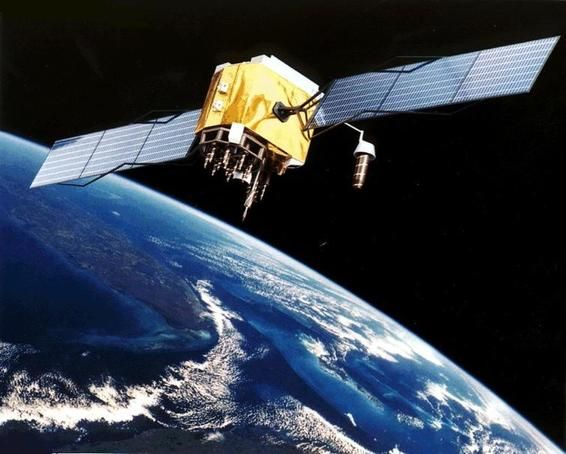Home Practice
For learners and parents For teachers and schools
Textbooks
Full catalogue
Pricing SupportLog in

We think you are located in United States. Is this correct?

# Chapter 5: Trigonometry

## 5.1 Introduction (EMA3M)

• Content covered in this chapter includes defining the trigonometric ratios and extending these definitions to any angle. Also covered is the definitions of the reciprocals of the trigonometric ratios. Both the trigonometric ratios and their reciprocals are solved for several special angles. In addition simple trigonometric equations are covered.
• Solving problems in two-dimensions using trigonometry is only covered later in the year and the content for this can be found in chapter 11.
• Similarity of triangles is fundamental to the trigonometric ratios
• Trigonometric ratios are independent of the lengths of the sides and instead depend only on the angles
• Doubling a ratio has a different effect from doubling an angle.
• Emphasise the value and importance of making sketches, where appropriate.
• Remind learners that angles in the Cartesian plane are always measured from the positive $$x$$-axis.
• When working with angles on the Cartesian plane remind learners to check that their answers are within the correct quadrant.
• Calculator skills are very important in this chapter. Methods for CASIO calculators are shown but practical demonstration may be required. For a SHARP calculator the keys are generally the same although the $$\(\keystroke{SHIFT}$$\) key is now the $$\(\keystroke{2ndF}$$\) key.
• We will refer to sine, cosine, tangent, secant, cosecant and cotangent as trigonometric ratios rather than as trigonometric functions. Both these terms are correct though but for the nature of the content in this chapter the term ratio better captures the content and is likely to be more accessible to learners at this stage.

Trigonometry deals with the relationship between the angles and sides of a triangle. We will learn about trigonometric ratios in right-angled triangles, which form the basis of trigonometry.

There are many applications of trigonometry. Of particular value is the technique of triangulation, which is used in astronomy to measure the distances to nearby stars, in geography to measure distances between landmarks, and in satellite navigation systems. GPS (the global positioning system) would not be possible without trigonometry. Other fields which make use of trigonometry include acoustics, optics, analysis of financial markets, electronics, probability theory, statistics, biology, medical imaging (CAT scans and ultrasound), chemistry, cryptology, meteorology, oceanography, land surveying, architecture, phonetics, engineering, computer graphics and game development.An artist's depiction of a GPS satellite orbiting the Earth. There are at least 24 GPS satellites operational at any one time. GPS uses an application of trigonometry, known as triangulation, to determine ones position. The accuracy of GPS is to within 15 metres.

Fabumaths has some useful links and content for trigonometry.

The following video covers a brief history of trigonometry and some of the uses of trigonometry.

Video: 2FN7

temp text